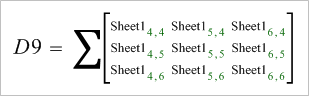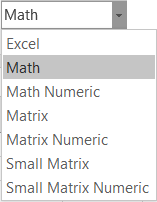## Change Style

Chooses how cell ranges are displayed.

### Usage

This formatting option applies to range of cells used in formulas. Generally it is impossible to display all values used in a range, so we have developed a variety of different style to suite differing needs and preferences.

Using the formula "=SUM(D4:F6)" as an example:

Mode Example Rendered Equations
Excel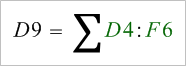Math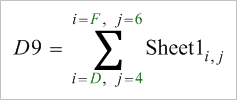Math Numeric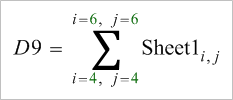Matrix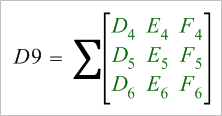Matrix Numeric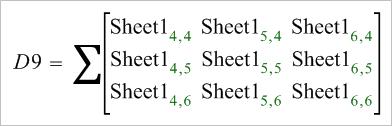Small Matrix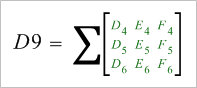Small Matrix Numeric